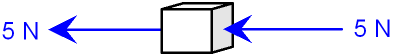gcsescience.com                                       15                                       gcsescience.com

Forces and Motion

Newton's Second Law of Motion - Unbalanced Forces.

What are Unbalanced Forces?

Forces are unbalanced when the resultant force is not zero.

What does Resultant Force mean?

Forces which act along a straight line can be
added if the forces are in the same direction
or
subtracted if the forces are in the opposite direction.

The force that you get after adding or subtracting is called
the resultant force. The resultant force is a single force
that has the same effect as all the other forces combined.In the free-body force diagram above, the forces
are in the same direction and can be added
producing a resultant force of 10 N pointing to the left.
This is an example of an
unbalanced force because the resultant force is not zero.

What does Newton's Second Law mean?

Newton's Second Law says that if the forces on
an object are unbalanced then the resultant force
will cause the motion of the object to change.
The bigger the force, the bigger the change in motion

A change in motion is called acceleration.

Newton's Second Law gives rise to the equation

Force = mass x acceleration.

F = m x a

This equation is important!  (continued on the next page).

gcsescience.com          Physics Quiz          Index          Force Quiz          gcsescience.com